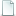## Global Synchronization for Discrete-Time Stochastic Complex Networks With Randomly Occurred Nonlinearities and Mixed Time Delays Zidong Wang and Yao Wang and Yurong LiuGlobal Synchronization for Discrete-Time Stochastic Complex Networks With Randomly Occurred Nonlinearities and Mixed Time Delays.pdf 923.80kB
Type: Paper
Tags: Computer-Assisted;Stochastic Processes;Time Factors

Bibtex:
```@ARTICLE{5342442,
author={Zidong Wang and Yao Wang and Yurong Liu},
journal={Neural Networks, IEEE Transactions on},
title={Global Synchronization for Discrete-Time Stochastic Complex Networks With Randomly Occurred Nonlinearities and Mixed Time Delays},
year={2010},
volume={21},
number={1},
pages={11-25},
abstract={In this paper, the problem of stochastic synchronization analysis is investigated for a new array of coupled discrete-time stochastic complex networks with randomly occurred nonlinearities (RONs) and time delays. The discrete-time complex networks under consideration are subject to: (1) stochastic nonlinearities that occur according to the Bernoulli distributed white noise sequences; (2) stochastic disturbances that enter the coupling term, the delayed coupling term as well as the overall network; and (3) time delays that include both the discrete and distributed ones. Note that the newly introduced RONs and the multiple stochastic disturbances can better reflect the dynamical behaviors of coupled complex networks whose information transmission process is affected by a noisy environment (e.g., Internet-based control systems). By constructing a novel Lyapunov-like matrix functional, the idea of delay fractioning is applied to deal with the addressed synchronization analysis problem. By employing a combination of the linear matrix inequality (LMI) techniques, the free-weighting matrix method and stochastic analysis theories, several delay-dependent sufficient conditions are obtained which ensure the asymptotic synchronization in the mean square sense for the discrete-time stochastic complex networks with time delays. The criteria derived are characterized in terms of LMIs whose solution can be solved by utilizing the standard numerical software. A simulation example is presented to show the effectiveness and applicability of the proposed results.},
keywords={Lyapunov methods;complex networks;delays;discrete time systems;linear matrix inequalities;nonlinear control systems;stochastic systems;Bernoulli white noise sequence;Lyapunov-like matrix functional;discrete-time network;free-weighting matrix method;linear matrix inequality technique;randomly occurred nonlinearities;stochastic complex networks;stochastic disturbance;stochastic synchronization analysis;time delays;Discrete time delays;distributed time delays;global synchronization;randomly occurred nonlinearity (RON);stochastic complex networks;stochastic coupling;Computer Simulation;Humans;Neural Networks (Computer);Nonlinear Dynamics;Reaction Time;Signal Processing, Computer-Assisted;Stochastic Processes;Time Factors},
doi={10.1109/TNN.2009.2033599},
ISSN={1045-9227},
month={Jan},}
```

Send Feedback